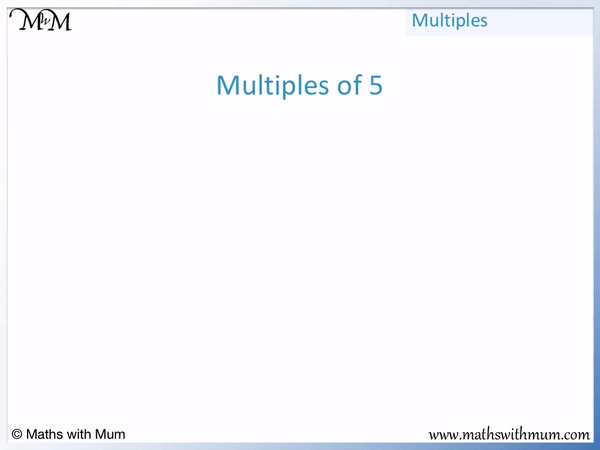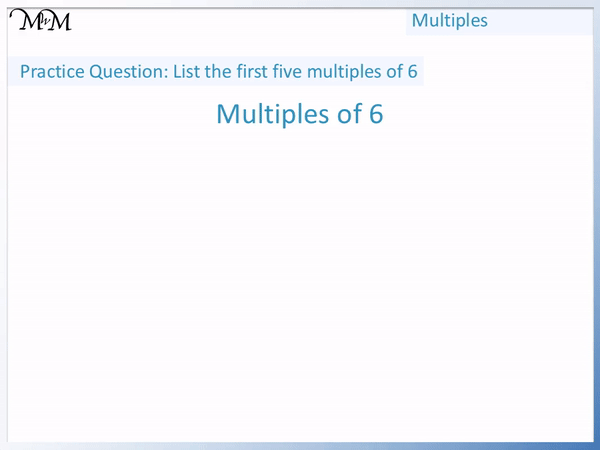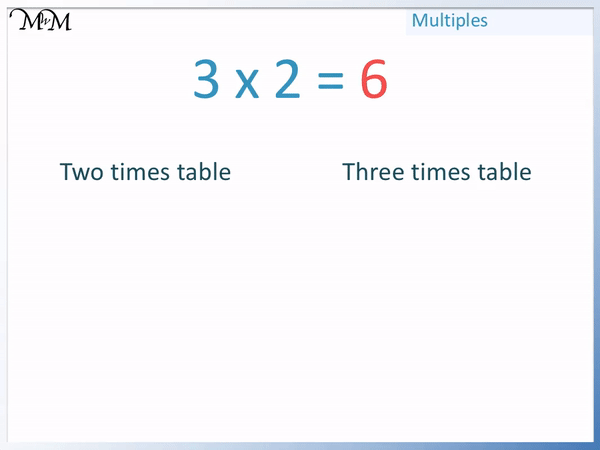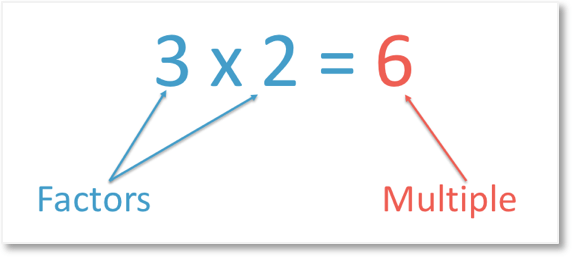# How to Find Multiples

How to Find Multiples• The multiples of a given number are the numbers that are in the times tables of that number.
• 1 × 5 = 5 and so, the first multiple of 5 is 5.
• 2 × 5 = 10 and so, the second multiple of 5 is 10.
• 3 × 5 = 15 and so, the third multiple of 5 is 15.
• 4 × 5 = 20 and so, the fourth multiple of 5 is 20.
• 5 × 5 = 25 and so, the fifth multiple of 5 is 25.
• There are an infinite number of multiples.
• For example the hundredth multiple of 5 is 500.
• The multiples of a number can be found by repeatedly adding that number.
• For example, 5, 10, 15, 20 and 25 are found by starting at 0 and adding 5.
To find a multiple of a number, multiply the number by any whole number.• The first few multiples of 6 are the numbers in the 6 times table.
• The multiples of 6 can be found by counting up in sixes starting from 0.
• 1 × 6 = 6 and so, the first multiple of 6 is 6.
• 2 × 6 = 12 and so, the second multiple of 6 is 12.
• 3 × 6 = 18 and so, the third multiple of 6 is 18.
• 4 × 6 = 24 and so, the fourth multiple of 6 is 24.
• 5 × 6 = 30 and so, the fifth multiple of 6 is 30.Supporting Lessons# Finding Multiples of Numbers

## What are Multiples?

Multiples are the numbers formed by multiplying a number by any other number. In simple terms, multiples of a given number are any numbers that are in the times tables of this number. For example, the multiples of 2 are 2, 4, 6, 8, 10, 12 and so on.

Many multiples are multiples of more than one number.

Here we can see that 6 is both a multiple of 2 and a multiple of 3.

This is because 2 × 3 = 6 and 3 × 2 = 6.6 is the third multiple of 2 and 6 is the second multiple of 3.

In any multiplication sentence, the numbers being multiplied together are the factors of the answer. This means that they divide exactly into the answer.In any multiplication sentence, the answer is a multiple of the numbers being multiplied together.## How to Find Multiples of Numbers

To find multiples of a number, multiply the number by any whole number. For example, 5 × 3 = 15 and so, 15 is the third multiple of 5.

For example, the first 5 multiples of 4 are 4, 8, 12, 16 and 20.1 × 4 = 4, therefore the 1st multiple of 4 is 4.

2 × 4 = 8, therefore the 2nd multiple of 4 is 8.

3 × 4 = 12, therefore the 3rd multiple of 4 is 12.

4 × 4 = 16, therefore the 4th multiple of 4 is 16.

5 × 4 = 20, therefore the 5th multiple of 4 is 20.We can see that the multiples of 4 increase by 4 each time.

To list multiples of a number, start at zero and keep adding this number. The multiples of any given number always have a difference between them that is equal to the given number. If you know a multiple of a number, you can find the next multiple by adding the number to it.

Here we will list the first five multiples of 6.

We can start from 0 and count up in sixes.

The first five multiples of 6 are 6, 12, 18, 24 and 30.We can see that 6 + 6 = 12, 12 + 6 = 18, 18 + 6 = 24 and 24 + 6 = 30.

We can also find the multiples of 6 by multiplying 6 by any number.

1 × 6 = 6, therefore the 1st multiple of 6 is 6.

2 × 6 = 12, therefore the 2nd multiple of 6 is 12.

3 × 6 = 18, therefore the 3rd multiple of 6 is 18.

4 × 6 = 24, therefore the 4th multiple of 6 is 24.

5 × 6 = 30, therefore the 5th multiple of 6 is 30.

In this next example, we will list the first five multiples of 8.

We can start at zero and count up in eights.

The first five multiples of 8 are 8, 16, 24, 32 and 40.

8 + 8 = 16, 16 + 8 = 24, 24 + 8 = 32 and 32 + 8 = 40.We can also find the multiples of 8 by multiplying 8 by any number.

1 × 8 = 8, therefore the 1st multiple of 8 is 8.

2 × 8 = 16, therefore the 2nd multiple of 8 is 16.

3 × 8 = 24, therefore the 3rd multiple of 8 is 24.

4 × 8 = 32, therefore the 4th multiple of 8 is 32.

5 × 8 = 40, therefore the 5th multiple of 8 is 40.

## Lists of Multiples

Here is a list of the first 12 multiples of the first 12 numbers:

The multiples of 1 are 1, 2, 3, 4, 5, 6, 7, 8, 9, 10, 11 and 12.

The multiples of 2 are 2, 4, 6, 8, 10, 12, 14, 16, 18, 20, 22 and 24.

The multiples of 3 are 3, 6, 9, 12, 15, 18, 21, 24, 27, 30, 33 and 36.

The multiples of 4 are 4, 8, 12, 16, 20, 24, 28, 32, 36, 40, 44 and 48.

The multiples of 5 are 5, 10, 15, 20, 25, 30, 35, 40, 45, 50, 55 and 60.

The multiples of 6 are 6, 12, 18, 24, 30, 36, 42, 48, 54, 60, 66 and 72.

The multiples of 7 are 7, 14, 21, 28, 35, 42, 49, 56, 63, 70, 77 and 84.

The multiples of 8 are 8, 16, 24, 32, 40, 48, 56, 64, 72, 80, 88 and 96.

The multiples of 9 are 9, 18, 27, 36, 45, 54, 63, 72, 81, 90, 99 and 108.

The multiples of 10 are 10, 20, 30, 40, 50, 60, 70, 80, 90, 100, 110 and 120.

The multiples of 11 are 11, 22, 33, 44, 55, 66, 77, 88, 99, 110, 121 and 132.

The multiples of 12 are 12, 24, 36, 48, 60, 72, 84, 96, 108, 120, 132 and 144.Now try our lesson on Multiplying by Multiples of 10 and 100 where we learn how to multiply by numbers in the 10 and 100 times table.error: Content is protected !!